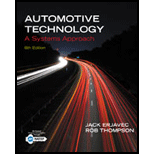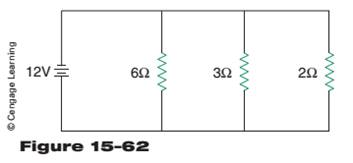Chapter 15, Problem 12RQAutomotive Technology: A Systems A...

6th Edition
Jack Erjavec + 1 other
ISBN: 9781133612315

Solutions

Chapter
SectionAutomotive Technology: A Systems A...

6th Edition
Jack Erjavec + 1 other
ISBN: 9781133612315
Textbook Problem

Solve the parallel circuit for total circuit resistance, total circuit amperage, and the amperage through each leg (Figure 15-62).To determine

The solutions of the given parallel circuit.

Explanation

Given:

Concept used:

Parallel circuits: When resistors are connected in parallel, the reciprocal of equivalent resistance is equal to the algebraic sum of the reciprocals of individual resistances.

1Req=1R1+1R2

Calculation:

The total circuit resistance of the circuit is,

1Req=1R1+1R2+1R3

Or, 1Req=16+13+12

Or, 1Req=1+2+36

Or, 1Req=<

Still sussing out bartleby?

Check out a sample textbook solution.

See a sample solution

The Solution to Your Study Problems

Bartleby provides explanations to thousands of textbook problems written by our experts, many with advanced degrees!

Get Started

Should Gladys call the legal authorities? Which agency should she call?

Principles of Information Security (MindTap Course List)

Database Systems: Design, Implementation, & Management

Why is it important to know the Greek alphabet?

Engineering Fundamentals: An Introduction to Engineering (MindTap Course List)

What are three typical reasons why companies develop their own information systems?

Systems Analysis and Design (Shelly Cashman Series) (MindTap Course List)

What is a mandatory access control?

Management Of Information Security